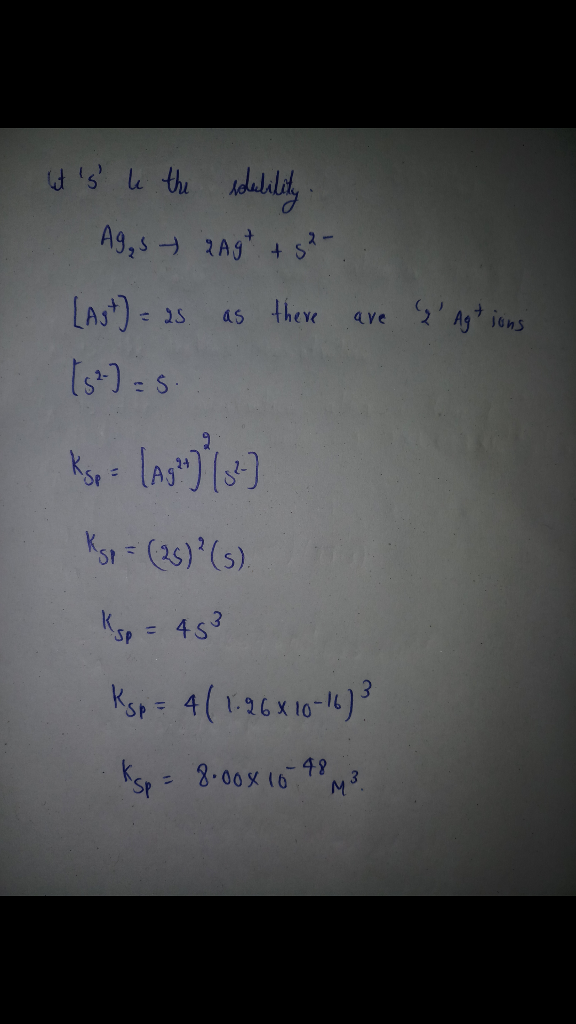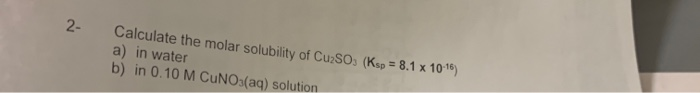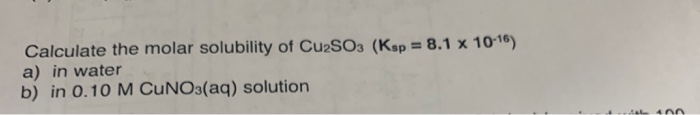# The molar solubility of Ag2S is 1.26 × 10-16 M in pure water. Calculate the Ksp for Ag2S.

The molar solubility of Ag2S is 1.26 × 10-16 M in pure water. Calculate the Ksp for Ag2S.##### Add Answer of: The molar solubility of Ag2S is 1.26 × 10-16 M in pure water. Calculate the Ksp for Ag2S.
Similar Homework Help Questions
• ### The molar solubility of ZnS is 1.6x10-12 M in pure water. Calculate the Ksp for ZnS.

The molar solubility of ZnS is 1.6x10-12 M in pure water. Calculate the Ksp for ZnS.

• ### The ksp of pbbr2 is 6.60 10 6. What is the molar solubility(M) of PbBr2 in pure water?

The Ksp of PbBr2 is 6.60*10^-6 What is the molar solubility(M) of PbBr2 in pure water? What is the molar solubility(M) of PbBr2 in 0.500M KBr solution? What is the molar solubility(M) of PbBr2 in a 0.500M Pb(NO3)2 solution?

• ### Calculate the molar solubility of CuSO. (Ksp = 8.1 x 10-16) a) in water b) in...Calculate the molar solubility of CuSO. (Ksp = 8.1 x 10-16) a) in water b) in 0.10 M CUNO3(aq) solution

• ### Determine the molar solubility of PbSO4 in pure water. Ksp (PbSO4) = 1.6 × 10-10. a) 4.12 × 10-5 M b) 1.35 × 10-4 M c) 4...

Determine the molar solubility of PbSO4 in pure water. Ksp (PbSO4) = 1.6 × 10-10. a) 4.12 × 10-5 M b) 1.35 × 10-4 M c) 4.89 × 10-4 M d) 1.26 × 10-5 M e) 1.28 × 10-8 M

• ### Calculate the molar solubility of Cu2SO3 (Ksp = 8.1 x 10-16) a) in water b) in...Calculate the molar solubility of Cu2SO3 (Ksp = 8.1 x 10-16) a) in water b) in 0.10 M CUNO3(aq) solution IL 4A

• ### Calculate the molar solubility of lead thiocyanate in pure water. The molar solubility is the maximum...

Calculate the molar solubility of lead thiocyanate in pure water. The molar solubility is the maximum amount of lead thiocyanate the solution can hold. Lead thiocyanate has a Ksp value of 2.0*10^-5.

• ### Use the Ksp values to calculate the molar solubility of each of the following compounds in pure...

Use the Ksp values to calculate the molar solubility of each of the following compounds in pure water.Part B: PbCl2 (Ksp = 1.17×10-5)FYI-Answer is not 1.43*10^-2 M

• ### Calculate Ksp for Ag2S(s), then calculate molar solubility of Ag+ and S2-. Delta G of Ag2S = -40.7KJ/mol Delta G of Ag+...

Calculate Ksp for Ag2S(s), then calculate molar solubility of Ag+ and S2-. Delta G of Ag2S = -40.7KJ/mol Delta G of Ag+ = 77.11KJ/mol Delta G of S2- = 83.7KJ/mol ICE table and clear breakdown of relationship of Ksp and Delta G. please.

• ### What is the molar solubility of silver chloride in pure water if its Ksp=1.8*10^-10

What is the molar solubility of silver chloride in pure water if its Ksp=1.8*10^-10

• ### Calculate Ksp for Ag2S(s), then using standard free energies of formation, calculate molar solubility of Ag+...

Calculate Ksp for Ag2S(s), then using standard free energies of formation, calculate molar solubility of Ag+ and S2-. Delta G of Ag2S = -40.7KJ/mol Delta G of Ag+ = 77.11KJ/mol Delta G of S2- = 83.7KJ/mol

Need Online Homework Help?